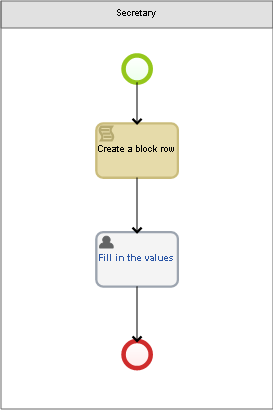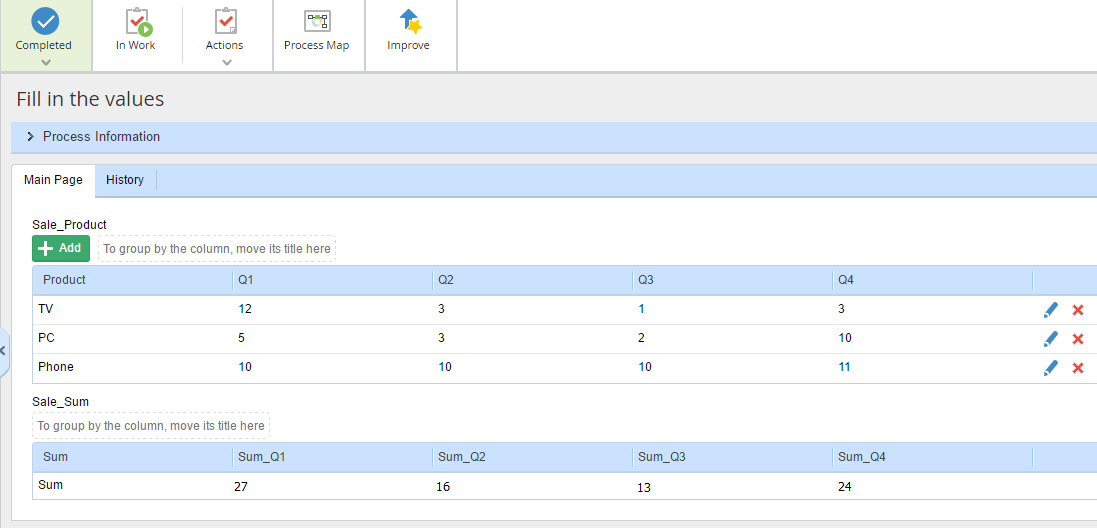# Dynamic forms of business process tasks: writing results of operations with block elements to another block

When you use blocks in process tasks, it may be necessary to write the variable values of one block as variable values of another block. For example, you many need to write the sum of values in a column of one block to another.

Say there are two blocks:

1) Sale_Product block

The block contains information about the sales volume of a company’s product for each quarter.

Block properties:

Product – product, String-type;

Q1 – sales volume of this product in the first quarter, Integer-type;
Q2 – sales volume of this product in the second quarter, Integer-type;
Q3 – sales volume of this product in the third quarter, Integer-type;
Q4 – sales volume of this product in the fourth quarter, Integer-type.

2) Sale_Sum block

The block contains information about the sales volume of all the products for each quarter. The block and its properties are read-only.

Block properties:

Sum – product, String-type;
Sum_Q1 – sales volume of all the products in the first quarter, Integer-type;
Sum_Q2 – sales volume of all the products in the second quarter, Integer-type;
Sum_Q3 – sales volume of all the products in the third quarter, Integer-type;
Sum_Q4 – sales volume of all the products in the fourth quarter, Integer-type.

Write a script, which will automatically fill in the values of the second unit when the values of the first unit are filled in. Use a standard on-change script, linked to a Sale_Product block variable. This script will be executed when a new element is added or an existing element is edited in the Sale_Product block. I.e. every time the Sale_Product block is changed, the values of the Sale_Sum block are recalculated.For the scripts to work you have to use the following namespace:

``using EleWise.ELMA.Model.Services;``

At first, when you open the task, the Sale_Sum block will be empty; therefore, you should add the row that will contain values of the sums:

``````public void Sum(Context context, EleWise.ELMA.Model.Views.FormViewBuilder<Context> form)
{
var newRow = InterfaceActivator.Create<P_Blok_Sale_Sum>();
//create a row of the Sale_Sum block
newRow.Sum_Q1 = 0; // assign the “0” value to the cells
newRow.Sum_Q2 = 0;
newRow.Sum_Q3 = 0;
newRow.Sum_Q4 = 0;
newRow.Sum = "Sum"; //assign the “Sum” value to the first cell
// add the created row to the Sale_Sum block
}
``````

Now sum up the values of the Sale_Product block and write the results to the Sale_Sum block. To pass values, use additional variables:

TotalSum_Q1 – sales volume of all the products in the first quarter, Integer-type;
TotalSum _Q2 – sales volume of all the products in the second quarter, Integer-type;
TotalSum _Q3 – sales volume of all the products in the third quarter, Integer-type;
TotalSum _Q4 – sales volume of all the products in the fourth quarter, Integer-type.

``````public void Sum_block(Context context, EleWise.ELMA.Model.Views.FormViewBuilder<Context> form)
{

context.TotalSum_Q1 = 0; // assign the “0” value to the context variables
context.TotalSum_Q2 = 0;
context.TotalSum_Q3 = 0;
context.TotalSum_Q4 = 0;

foreach (var item in context. Sale_Product)
{
if(item.Q1 == null) // check, if the cell is filled in; if it is not,
assign the “0”value
item.Q1 = 0;
if(item.Q2 == null)
item.Q2 = 0;
if(item.Q3 == null)
item.Q3 = 0;
if(item.Q4 == null)
item.Q4 = 0;

context.TotalSum_Q1 += item.Q1;
//add the value of the Sale_Product block cell to the context variable
context.TotalSum_Q2 += item.Q2;
context.TotalSum_Q3 += item.Q3;
context.TotalSum_Q4 += item.Q4;
}

foreach (var item in context.Sale_Sum.ToList())
{
item.Sum_Q1 = context.TotalSum_Q1;
//write the context variable values to the Sale_Sum block cells
item.Sum_Q2 = context.TotalSum_Q2;
item.Sum_Q3 = context.TotalSum_Q3;
item.Sum_Q4 = context.TotalSum_Q4;
}
``````

Use a simple process to see how it will be displayed in Web Application.When you open the “Fill in the values” task, a row of the Sale_Sum block is already created and assigned with default values:After adding rows to the Sale_Product block, the values of the Sale_Sum block are automatically recalculated:It is convenient when one user inputs current values in a process, and another user needs to be shown only the aggregated values.